Courses

# Volumetric Properties of Mixtures Civil Engineering (CE) Notes | EduRev

## Civil Engineering (CE) : Volumetric Properties of Mixtures Civil Engineering (CE) Notes | EduRev

The document Volumetric Properties of Mixtures Civil Engineering (CE) Notes | EduRev is a part of the Civil Engineering (CE) Course Thermodynamics.
All you need of Civil Engineering (CE) at this link: Civil Engineering (CE)

Volumetric Properties of Mixtures

The EOSs discussed in the preceding sections may be applied to mixtures as well, through use of what are called “mixing rules”. Such rules help re-define the fundamental parameters of each type of EOS in terms of those corresponding to pure species and overall composition. The principle behind these rules is that the mixture parameters are equivalent to those of a “hypothetical” pure species, which would display the same behaviour as the mixture.  They are essentially semi-empirical in nature, in that they contain parameters which although grounded in molecular theory are difficult to predict fully. Nevertheless, such rules have proved reasonably reliable for prediction of mixture properties for most practical, engineering applications.

Virial EOS

For a gas mixture the virial EOS is exactly the same as for a pure species (eqn. 2.12):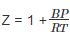However, for the mixture the second virial coefficient ‘B’ is dependent not only on temperature but also on the mixture composition. Its exact composition dependence is derivable from the relations provided by statistical mechanics, and takes the following form: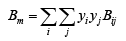...(2.26)

Where, y i = mole fractions in a gas mixture. The indices i and j identify species, and both run over all species present in the mixture. The virial coefficient B ij characterizes a bimolecular interaction between molecule i and molecule j, and therefore B ij = B ji. The summation in eqn. 2.26 accounts for all possible bimolecular interactions. For a binary mixture i = 2 and j = 2; the expansion of eqn.2.23 yields:

B = y21B11 + 2 y1 yB12 +y22B22                          ...(2.27)

Since they correspond to pure species, the calculation of the parameters B 11 and B 22 can be made directly employing eqns. 2.13 – 2.15. For any cross-coefficient B ij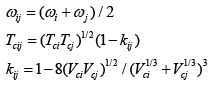Often  is set to zero for simplicity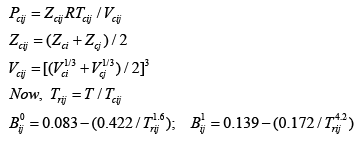Finally: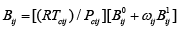...(2.28)

Next B m is computed by eqn. 2.26, which is used in eqn. 2.12 for computing the mixture molar volume.

Cubic EOS: The parameters in all cubic EOSs are principally: a, b and ω. It follows that for computing the molar volume of a mixture these parameters need to be re-defined using mixing rules. For a binary mixture they are: For a binary mixture (m):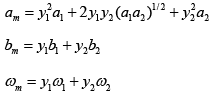The values of each parameters can be computed after those of the individual species are calculated using the expressions that apply to each type of cubic EOS (see table 2.1).

Generalized Correlations Generalized correlations presented above for pure species may also be conveniently extended to prediction of volumetric properties of gas phase mixtures as well. For the mixture, the apparent critical properties are computed using the following set of linear relations: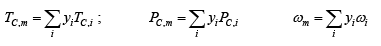...(2.32)

The subscript ‘i’ runs over all the species present in the mixture ‘m’. The above properties are designated as “pseudo-critical” as they do not represent the true critical properties of a mixture; indeed the latter are most often difficult to obtain. The pseudo-reduced temperature and pressure, are then determined by: Tr ,m = T /TC,m ; Pr ,m = P / PC,m . As for pure components, the compressibility factor for the mixture is next obtained using standard functions of Z0 (Tr Pr) andZ0 (Tr Pr)  which are then used in the following equation:  Z=Z0m + ω Z 1m                                 ...(2.33)

Offer running on EduRev: Apply code STAYHOME200 to get INR 200 off on our premium plan EduRev Infinity!

## Thermodynamics

56 videos|92 docs|33 tests

,

,

,

,

,

,

,

,

,

,

,

,

,

,

,

,

,

,

,

,

,

;